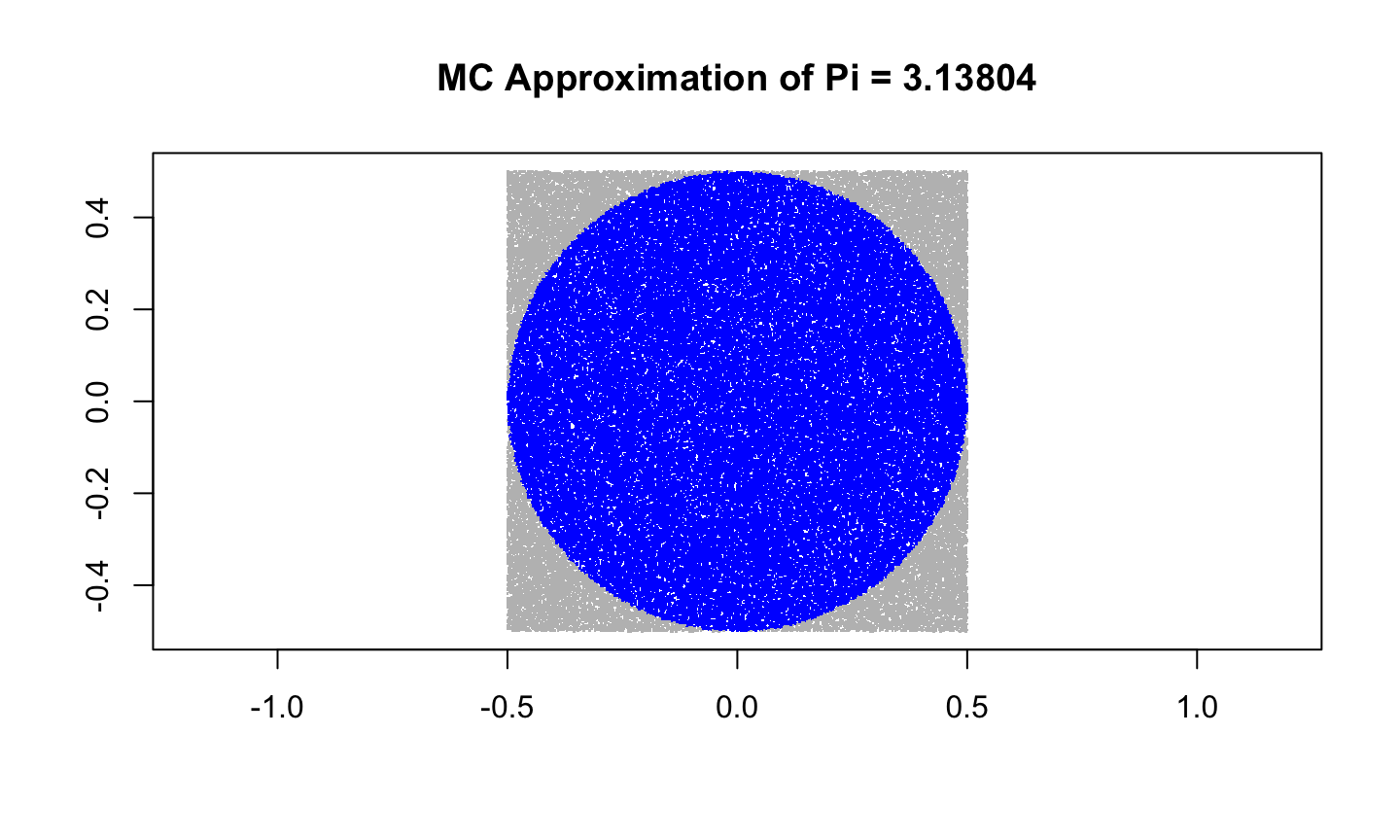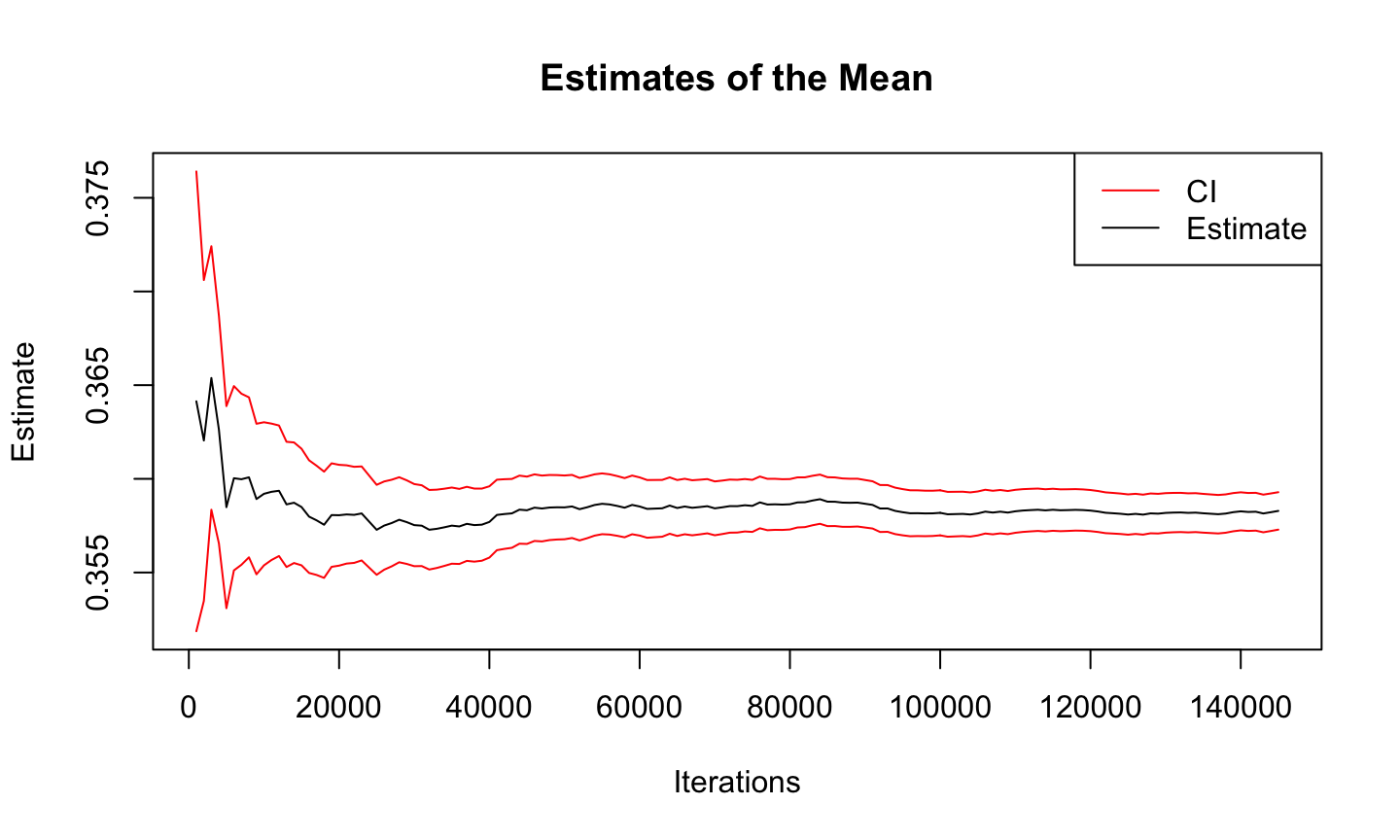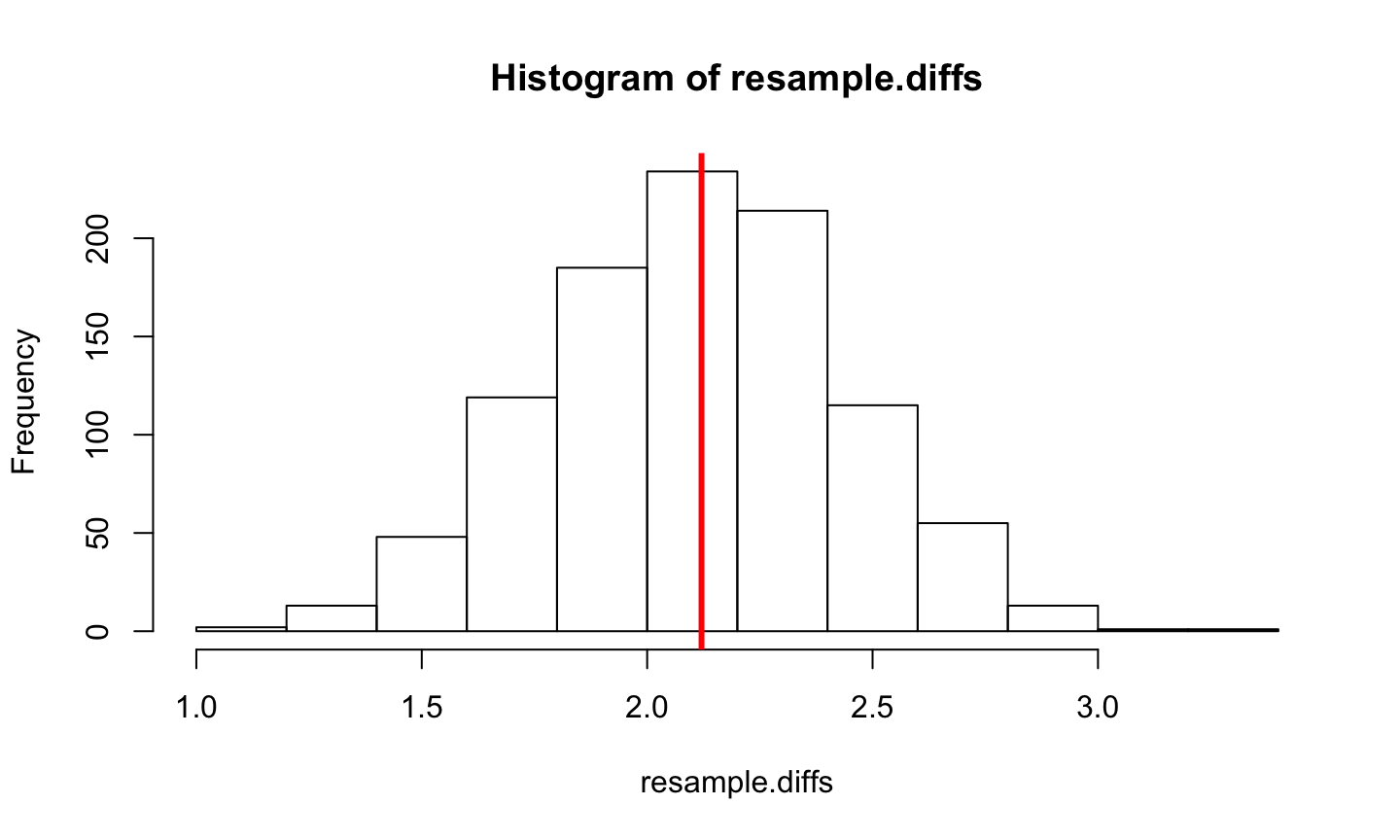## Agenda

• Ordinary Monte Carlo
• Examples
• Monte Carlo integration
• Bootstrap
• Toy collector exercise

## Ordinary Monte Carlo

The “Monte Carlo method” refers to the theory and practice of learning about probability distributions by simulation rather than calculus. In ordinary Monte Carlo (OMC) we use IID simulations from the distribution of interest. Suppose $$X_1, X_2, \dots$$ are IID simulations from some distribution, and suppose we want to know an expectation $\theta = E\left[ Y_1 \right] = E\left[ g(X_1) \right].$ The law of large numbers (LLN) then says $\bar{y}_n = \frac{1}{n} \sum_{i=1}^n Y_i = \frac{1}{n} \sum_{i=1}^n g(X_i)$ converges in probability to $$\theta$$.

## Ordinary Monte Carlo

The central limit theorem (CLT) says $\frac{\sqrt{n} (\bar{y}_n - \theta) }{\sigma} \stackrel{d}{\rightarrow} \mbox{N}(0,1) .$ That is, for sufficiently large $$n$$, $\bar{y}_n \sim \mbox{N}( \theta , \sigma^2 / n).$ Further, we can estimate the standard error $$\sigma / \sqrt{n}$$ with $$s_n / \sqrt{n}$$ where $$s_n$$ is the sample standard deviation.

## Ordinary Monte Carlo

We can also use the CLT form a confidence interval with $Pr( \bar{y}_n - 1.96 s_n / \sqrt{n} < \mbox{E} Y_1 < \bar{y}_n + 1.96 s_n / \sqrt{n} ) \approx 0.95 .$ Or we could simulate until a half-width (or width) of this confidence interval is sufficiently small, say less than $$\epsilon>0$$. That is, simulate until $1.96 s_n / \sqrt{n} < \epsilon .$

## Ordinary Monte Carlo

The theory of OMC is just the theory of frequentist statistical inference. The only differences are that

• the “data” $$X_1, \dots, X_n$$ are computer simulations rather than measurements on objects in the real world
• the “sample size” $$n$$ is the number of computer simulations rather than the size of some real world data
• the unknown parameter $$\theta$$ is in principle completely known, given by some integral, which we are unable to do.

## Ordinary Monte Carlo

Everything works just the same when the data $$X_1, X_2, \dots$$, which are computer simulations are vectors. But the functions of interest $$g(X_1), g(X_2), \dots$$ are scalars.

OMC works great, but it can be very difficult to simulate IID simulations of random variables or random vectors whose distribution is not brand name distributions

## Approximating the Binomial

Suppose we flip a coin 10 times and we want to know the probability of getting more than 3 heads. This is trivial for the Binomial distribution, which we'll ignore.

runs <- 10000
one.trial <- function(){
sum(sample(c(0,1),10,replace=T)) > 3
}
mc.binom <- sum(replicate(runs,one.trial()))/runs
mc.binom
##  0.8233
pbinom(3,10,0.5,lower.tail=FALSE)
##  0.828125

Exercise: Program this example and estimate the Monte Carlo standard error

## Aproximating $$\pi$$

• Area of a circle is $$\pi r^2$$
• If we draw a square containing that circle its area will be $$4r^2$$
• So the ratio of the area of the circle to the area of the square is $\frac{\pi r^2}{4r^2} = \frac{\pi}{4}$
• Given this fact, we can empirically determine the ratio of the area of the circle to the area of the square we can simply multiply this number by 4 and we'll get our approximation of $$\pi$$.
• How?

## Aproximating $$\pi$$

• Randomly sample $$x$$ and $$y$$ values on the unit square centered at 0
• If $$x^2 + y^2 \le .5^2$$ then the point is in the circle
• The ratio of points in the circle multiplied by 4 is our estimate of $$\pi$$
runs <- 100000
xs <- runif(runs,min=-0.5,max=0.5)
ys <- runif(runs,min=-0.5,max=0.5)
in.circle <- xs^2 + ys^2 <= 0.5^2
mc.pi <- (sum(in.circle)/runs)*4

## Aproximating $$\pi$$

plot(xs,ys,pch='.',col=ifelse(in.circle,"blue","grey")
,xlab='',ylab='',asp=1,
main=paste("MC Approximation of Pi =",mc.pi))## Example: Integration

Let $$X \sim \Gamma (3/2, 1)$$, i.e.
$f(x) = \frac{2}{\sqrt{\pi}} \sqrt{x} e^{-x} I(x>0) .$ Suppose we want to find \begin{aligned} \theta & = \mbox{E} \left[ \frac{1}{(X+1)\log (X+3)} \right]\\ & = \int_{0}^{\infty} \frac{1}{(x+1)\log (x+3)} \frac{2}{\sqrt{\pi}} \sqrt{x} e^{-x} dx . \end{aligned}

• The expectation (or integral) $$\theta$$ is intractable, we don't know how to compute it analytically
• Further, suppose we want to estimate this quantity such that a 95% CI length is less than 0.002

## Example: Integration

n <- 1000
x <- rgamma(n, 3/2, scale=1)
mean(x)
##  1.472507
y <- 1/((x+1)*log(x+3))
est <- mean(y)
est
##  0.3641365
mcse <- sd(y) / sqrt(length(y))
interval <- est + c(-1,1)*1.96*mcse
interval
##  0.3518669 0.3764061

## Example: Sequential stopping rule

eps <- 0.002
len <- diff(interval)
plotting.var <- c(est, interval)
while(len > eps){
new.x <- rgamma(n, 3/2, scale=1)
new.y <- 1/((new.x+1)*log(new.x+3))
y <- cbind(y, new.y)
est <- mean(y)
mcse <- sd(y) / sqrt(length(y))
interval <- est + c(-1,1)*1.96*mcse
len <- diff(interval)
plotting.var <- rbind(plotting.var, c(est, interval))
}
list(interval, length(y))
## []
##  0.3572893 0.3592867
##
## []
##  145000
temp <- seq(1000, length(y), 1000)

## Example: Sequential stopping rule

plot(temp, plotting.var[,1], type="l", ylim=c(min(plotting.var), max(plotting.var)),
main="Estimates of the Mean", xlab="Iterations", ylab="Estimate")
points(temp, plotting.var[,2], type="l", col="red")
points(temp, plotting.var[,3], type="l", col="red")
legend("topright", legend=c("CI", "Estimate"), lty=c(1,1), col=c(2,1))## Permutations with sample()

• sample() is powerful – it works on any object that has a defined length().
• Permutations
sample(5)
##  1 4 2 3 5
sample(1:6)
##  2 1 3 6 5 4
replicate(3,sample(c("Curly","Larry","Moe","Shemp")))
##      [,1]    [,2]    [,3]
## [1,] "Larry" "Moe"   "Shemp"
## [2,] "Shemp" "Larry" "Curly"
## [3,] "Curly" "Shemp" "Moe"
## [4,] "Moe"   "Curly" "Larry"

## Resampling with sample()

Resampling from any existing distribution gives bootstrap estimators

bootstrap.resample <- function (object) sample (object, length(object), replace=TRUE)
replicate(5, bootstrap.resample (6:10))
##      [,1] [,2] [,3] [,4] [,5]
## [1,]    9    6    7    6   10
## [2,]    8    8    8    6    9
## [3,]   10   10    9    7    7
## [4,]   10    6    9    6    7
## [5,]    7    6    8    6    6

Recall: the jackknife removed one point from the sample and recalculated the statistic of interest. Here we resample the same length with replacement.

## Bootstrap test

The 2-sample t-test checks for differences in means according to a known null distribution. Let's resample and generate the sampling distribution under the bootstrap assumption:

library(MASS)
diff.in.means <- function(df) {
mean(df[df$Sex=="M","Hwt"]) - mean(df[df$Sex=="F","Hwt"])
}
resample.diffs <- replicate(1000, diff.in.means(cats[bootstrap.resample(1:nrow(cats)),]))

## Bootstrap test

hist(resample.diffs); abline(v=diff.in.means(cats), col=2, lwd=3)## Summary

• Ordinary Monte Carlo
• Repeated random sampling to obtain numerical results
• Using randomness to solve problems
• Most useful when it is difficult or impossible to use other approaches
• Can you solve The Riddler?

## Exercise: Toy Collector

Children (and some adults) are frequently enticed to buy breakfast cereal in an effort to collect all the action figures. Assume there are 15 action figures and each cereal box contains exactly one with each figure being equally likely.

• Find the expected number of boxes needed to collect all 15 action figures.
• Find the standard deviation of the number of boxes needed to collect all 15 action figures.
• Now suppose we no longer have equal probabilities, instead let
Figure A B C D E F G H I J K L M N O
Probability .2 .1 .1 .1 .1 .1 .05 .05 .05 .05 .02 .02 .02 .02 .02
• Estimate the expected number of boxes needed to collect all 15 action figures.
• What is the uncertainty of your estimate?
• What is the probability you bought more than 50 boxes? 100 boxes? 200 boxes?